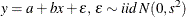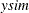# The MODEL Procedure

### Example 19.15 Simulated Method of Moments—Simple Linear Regression

This example illustrates how to use SMM to estimate a simple linear regression model for the following process:In the following SAS statements,is simulated, and the first moment and the second moment ofare compared with those of the observed endogenous variable y.

title "Simple regression model";

data regdata;
do i=1 to 500;
x = rannor( 1013 );
Y = 2 + 1.5 * x + 1.5 * rannor( 1013 );
output;
end;
run;

proc model data=regdata;
parms a b s;
instrument x;

ysim = (a+b*x) + s * rannor( 8003 );
y = ysim;
eq.ysq = y*y - ysim*ysim;

fit y ysq / gmm ndraw;
bound s > 0;
run;


Alternatively, the MOMENT statement can be used to specify the moments using the following syntax:

proc model data=regdata;
parms a b s;
instrument x;

ysim = (a+b*x) + s * rannor( 8003 );
y = ysim;
moment y = (2);

fit y / gmm ndraw;
bound s > 0;
run;


The output of the MODEL procedure is shown in Output 19.15.1:

Output 19.15.1: PROC MODEL Output

 Simple regression model

The MODEL Procedure

Model Summary
Model Variables 1
Parameters 3
Equations 2
Number of Statements 4

Model Variables Y a b s ysq Y

The 2 Equations to Estimate
Y = F(a(1), b(x), s)
ysq = F(a, b, s)
Instruments 1 x

Nonlinear GMM Parameter Estimates
Parameter Estimate Approx Std Err t Value Approx
Pr > |t|
a 2.065983 0.0657 31.45 <.0001
b 1.511075 0.0565 26.73 <.0001
s 1.483358 0.0498 29.78 <.0001